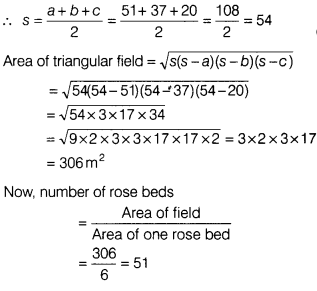# The sides of a triangular field are 51m, 37 m and 20 m. Find the number of rose beds

The sides of a triangular field are 51m, 37 m and 20 m. Find the number of rose beds that can be prepared in the field, if each rose bed occupies a space of 6 sq m.

Given, sides if a triangular field are 51 m,37 m and 20 m,
Let a=51 m , b=37 m, c=20 m